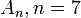# Difference between revisions of "Alternating group:A7"

View a complete list of particular groups (this is a very huge list!)[SHOW MORE]

## Definition

This group is defined as the alternating group of degree$7$, i.e., the alternating group on a set of size$7$. In other words, it is the subgroup of symmetric group:S7 comprising the even permutations.

## Arithmetic functions

### Basic arithmetic functions

Want to compare and contrast arithmetic function values with other groups of the same order? Check out groups of order 2520#Arithmetic functions
Function Value Similar groups Explanation
order (number of elements, equivalently, cardinality or size of underlying set) 2520 groups with same order As alternating group$A_n, n = 7$:$n!/2 = 7!/2 = = (7 \cdot 6 \cdot 5 \cdot 4 \cdot 3 \cdot 2 \cdot 1)/2 = 2520$
Template:Arithmetic function given order
derived length -- not a solvable group
nilpotency class -- not a nilpotent group
Frattini length 1 groups with same order and Frattini length | groups with same Frattini length Frattini-free group: intersection of all maximal subgroups is trivial
minimum size of generating set 2 groups with same order and minimum size of generating set | groups with same minimum size of generating set

### Arithmetic functions of a counting nature

Function Value Explanation
number of subgroups 3786 See subgroup structure of alternating group:A7, subgroup structure of alternating groups
number of conjugacy classes 9 See element structure of alternating group:A7, element structure of alternating groups
number of conjugacy classes of subgroups 40 See subgroup structure of alternating group:A7, subgroup structure of alternating groups